Technical Article

# Robot Manipulation Control with Inverse and Forward Kinematics

April 23, 2021 by Anish Devasia

## Learn how to control robotic arms more concisely with forward and inverse robotic kinematics techniques.

#### What is the Kinematic Chain?

Donald L. Pieper invented the 321 kinematic chain. It is used in most robotic arms with high Degrees of Freedom (DoF) and is made of intersecting revolute joints.

The Stanford arm was one of the first models of this design. It had six degrees of freedom from two revolute joints, a prismatic joint and a spherical joint. Most robotic arms used in industry are advanced iterations of the Stanford arm.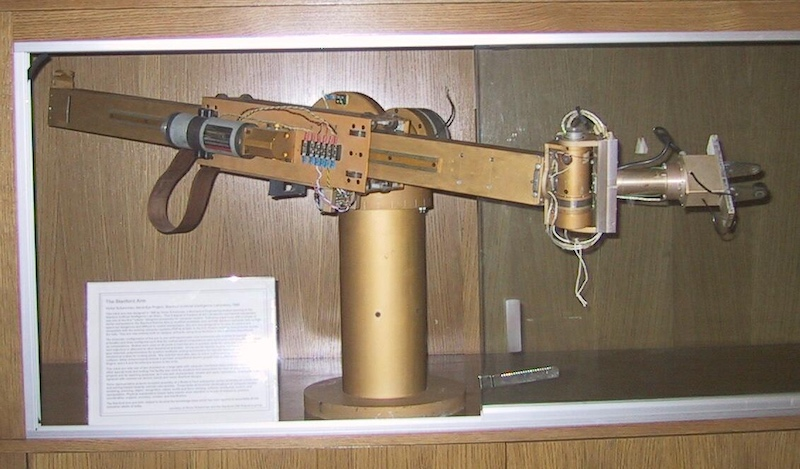##### The Stanford arm. Image used courtesy of Stanford University

An engineer can use forward kinematics and inverse kinematics to figure out the positions of end effectors and manipulate the robotic arm.

### Forward Kinematics

In any kinematic chain, from the base of the robotic arm, different links are serially connected with revolute links or prismatic joints. The end effector or tooltip is attached at the end of the last link. Forward kinematics can calculate the position and orientation of the end effector with joint variables.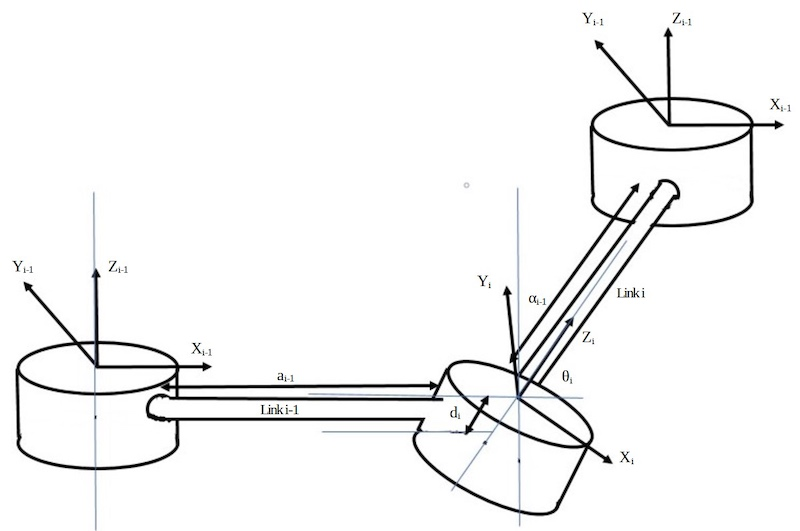##### Figure 1: Representative image of kinematic chain showing Denavit Hartenberg parameters and reference frames. Information used courtesy of S. Kucuk and Z. Bingul

The Denavit-Hartenberg method is the commonly used method for the forward kinematics model. It uses four parameters at each axis to define a system. They are:

• ai-1 representing the link length
• αi-1 denotes the link twist
• di stands for link offset
• θi for the joint angle

Denavit-Hartenberg’s model is used when there are multiple reference frames in a system. Different reference frames can be placed at each joint. Zi is pointing in the direction of rotation at the joints or the direction in which the joint slides to and from. Denavit-Hartenberg parameters are decided after the axes of coordinate frames are determined at each joint.

Suppose coordinate frames and DH parameters are clearly marked. The link length ai-1 is measured as the length between the axes Zi and Zi-1 along the Xi-1 axis. αi-1 is the angle between the same two Z axes measured along the Xi axis.

di is the distance between Xi-1 and Xi measured along the Zi axis, and θi is the angle between Xi-1 and Xi. The general Transformation matrix Tii-1 for a link can be written as follows.

Tii-1=Rx(ai-1) Dx(ai-1)Rzi)Qi(di)

Similarly, the transformation matrix for the reference frames at each joint can be calculated, TT01, T12,... The transformation matrix of the end effector in relation to the base can be calculated by multiplying the transformation matrix at each joint.

Tbaseend-effector =  T01T12...Tn-1n

This transformation matrix will give px, py, and pz, the position coordinates of the end-effector.

### Inverse Kinematics

Calculating the position of the end-effector with forward kinematics is not sufficient for controlling robotic arms. We need to calculate how to reach the desired state of the arm from the current state by reconfiguring the kinematic links. The calculations for it are referred to as inverse kinematics.

Inverse kinematics can be solved using the geometric solution approach or the algebraic solution approach. Let's take a brief look at the two approaches.

### Geometric Solution Approach

In this method, the joint space is divided into multiple two-dimensional planes and trigonometrically finding solutions in each plane.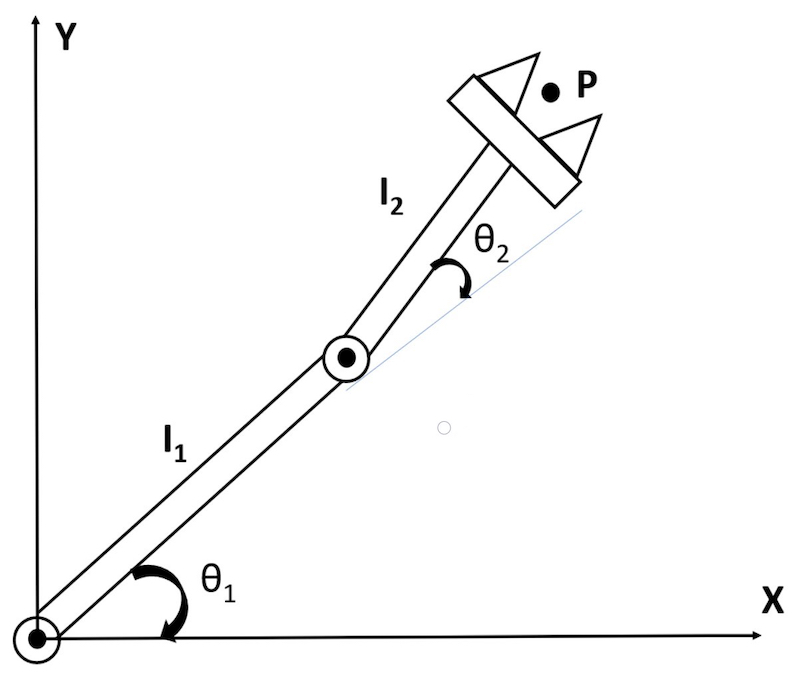##### Figure 2: For example, consider a manipulator with two degrees of freedom. Information used courtesy of S. Kucuk and Z. Bingul

This can be simplified to a simple geometric problem, as shown below.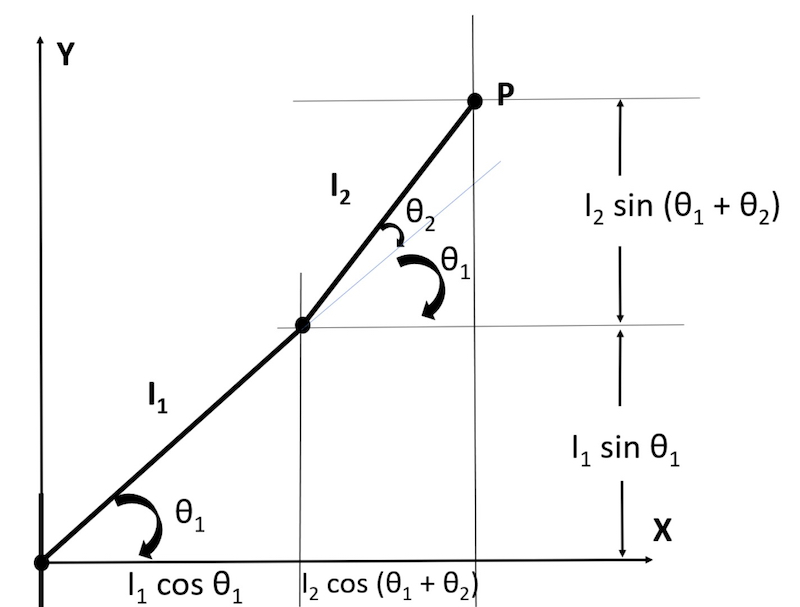##### Figure 3: Now, simple geometry can be applied to calculate the parameters. Information used courtesy of S. Kucuk and Z. Bingul

However, it gets far too complex and time-consuming to calculate when robotic arms with higher degrees of freedoms are involved.

In some robotic arms, the manipulator only operates in two dimensions. In other robotic arms, it operates in three-dimensional space. Keeping track of the different two-dimensional planes with respect to each other becomes tedious with a higher number of joints.

### Algebraic Solution Approach

In forward kinematics, the transformation matrix for the end effector to the base is found by multiplying all the transformation matrices at joints.

Tbaseend-effector =  T01T12...Tn-1n

For six-axis robots with six degrees of freedom, the equation becomes:

T0=T01T12T23T34T45T56

To find the inverse kinematic solution for the first joint, the inverse matrix of the transformation matrix T01 (which is [T01]-1) to both sides of the equation above.

[T01]-1T06 = [T01]-1T01T12T23T34T45T56

For a robotic arm with six degrees of freedom, 12 algebraic equations need to be solved to get the joint space parameters of one joint. Similarly, the joint space parameters for all six joints can be found.

It takes a long time to solve it manually. Today with access to advancing computing power, algorithms can solve it in a fraction of a second. This makes it simpler than the geometric solution approach in modern-day applications.

For a kinematic chain, forward kinematics is to calculate the end-effector position in relation to the base. The Denavit-Hartenberg parameters are used for mathematical calculations as it has variables to represent rotational and translational parameters.

The transformation matrix for each joint can be individually calculated and multiplied to obtain the Tend-effectorbase. This final matrix gives the position coordinates of the end effector.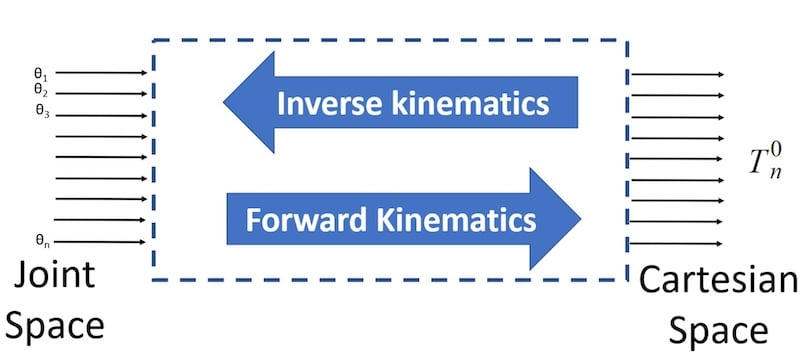##### Figure 4: Inverse kinematics is used to find the joint space parameters when Cartesian parameters are known. This is essential to manipulate the end effect by inducing different movements to the different joints.

In simple terms, forward kinematics is used to convert variables in joint space (Denavit-Hartenberg parameters) to Cartesian coordinates, and inverse kinematics is used for the reverse operation.
Using these kinematic methods can help engineers understand how to control robot arms more carefully.